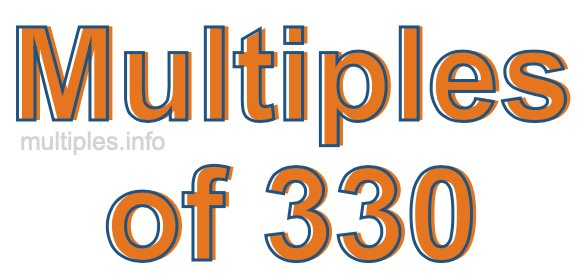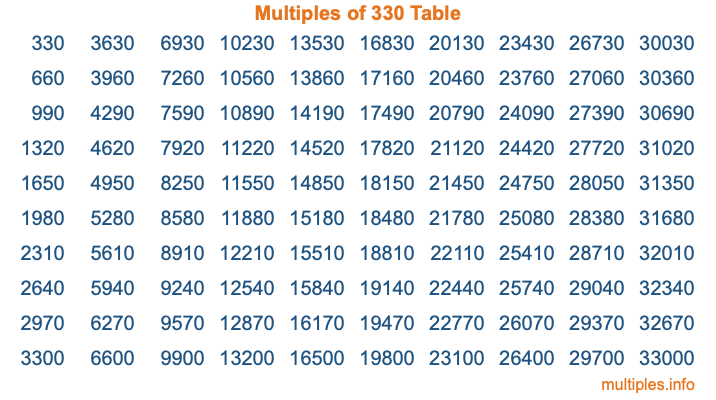Multiples of 330Welcome to the Multiples of 330 page. Here we will first teach you everything you will ever need to know about the multiples of 330, and then give you a study guide summary of everything we taught you to make sure you remember it all. Use this page to look up facts and learn information about the multiples of 330. This page will make you a multiples of three hundred thirty expert!

Definition of Multiples of 330
Multiples of 330 are all the numbers that when divided by 330 equal an integer. Each of the multiples of 330 are called a multiple. A multiple of 330 is created by multiplying 330 by an integer.

Therefore, to create a list of multiples of 330, you start with 1 multiplied by 330, then 2 multiplied by 330, then 3 multiplied by 330, and so on for as long as you want. Thus, the list of the first five multiples of 330 is 330, 660, 990, 1320, and 1650. To see a larger list of multiples of 330, see the printable image of Multiples of 330 further down on this page. We also have a category where you can choose any nth multiple of 330.

Multiples of 330 Checker
The Multiples of 330 Checker below checks to see if any number of your choice is a multiple of 330. In other words, it checks to see if there is any number (integer) that when multiplied by 330 will equal your number. To do that, we divide your number by 330. If the the quotient is an integer, then your number is a multiple of 330.

Is  a multiple of 330?

Least Common Multiple of 330 and ...
A Least Common Multiple (LCM) is the lowest multiple that two or more numbers have in common. This is also called the smallest common multiple or lowest common multiple and is useful to know when you are adding our subtracting fractions. Enter one or more numbers below (330 is already entered) to find the LCM.

Check out our LCM Calculator if you need more details about the Least Common Multiple or if you need the LCM for different numbers for adding and subtraction fractions.

nth Multiple of 330
As we stated above, 330 is the first multiple of 330, 660 is the second multiple of 330, 990 is the third multiple of 330, and so on. Enter a number below to find the nth multiple of 330.

th multiple of 330

Multiples of 330 vs Factors of 330
330 is a multiple of 330 and a factor of 330, but that is where the similarities end. All postive multiples of 330 are 330 or greater than 330. All positive factors of 330 are 330 or less than 330.

Below is the beginning list of multiples of 330 and the factors of 330 so you can compare:

Multiples of 330: 330, 660, 990, 1320, 1650, etc.

Factors of 330: 1, 2, 3, 5, 6, 10, 11, 15, 22, 30, 33, 55, 66, 110, 165, 330

As you can see, the multiples of 330 are all the numbers that you can divide by 330 to get a whole number. The factors of 330, on the other hand, are all the whole numbers that you can multiply by another whole number to get 330.

It's also interesting to note that if a number (x) is a factor of 330, then 330 will also be a multiple of that number (x).

Multiples of 330 vs Divisors of 330
The divisors of 330 are all the integers that 330 can be divided by evenly. Below is a list of the divisors of 330.

Divisors of 330: 1, 2, 3, 5, 6, 10, 11, 15, 22, 30, 33, 55, 66, 110, 165, 330

The interesting thing to note here is that if you take any multiple of 330 and divide it by a divisor of 330, you will see that the quotient is an integer.

Multiples of 330 Table
Below is an image of the first 100 multiples of 330 in a table. The table is in chronological order, column by column. The first column has the first ten multiples of 330, the second column has the next ten multiples of 330, and so on.The Multiples of 330 Table is also referred to as the 330 Times Table or Times Table of 330. You are welcome to print out our table for your studies.

Negative Multiples of 330
Although not often discussed or needed in math, it is worth mentioning that you can make a list of negative multiples of 330 by multiplying 330 by -1, then by -2, then by -3, and so on, to get the following list of negative multiples of 330:

-330, -660, -990, -1320, -1650, etc.

Multiples of 330 Summary
Below is a summary of important Multiples of 330 facts that we have discussed on this page. To retain the knowledge on this page, we recommend that you read through the summary and explain to yourself or a study partner why they hold true.

There are an infinite number of multiples of 330.

A multiple of 330 divided by 330 will equal a whole number.

330 divided by a factor of 330 equals a divisor of 330.

The nth multiple of 330 is n times 330.

The largest factor of 330 is equal to the first positive multiple of 330.

330 is a multiple of every factor of 330.

330 is a multiple of 330.

A multiple of 330 divided by a divisor of 330 equals an integer.

330 divided by a divisor of 330 equals a factor of 330.

Any integer times 330 will equal a multiple of 330.

Multiples of a Number
Here you can get the multiples of another number, all with the same attention to detail as we did for multiples of 330 on this page.

Multiples of
Multiples of 331
Did you find our page about multiples of three hundred thirty educational? Do you want more knowledge? Check out the multiples of the next number on our list!